# Sample Data: Longleaf Pines

Locations of longleaf pine trees annotated with diameter at breast height (in centimeters) marks

## Details

Locations of longleaf pine trees in the observation region Rectangle[{0, 0}, {200, 200}] meters, annoatated with diameter at breast height (in centimeters) marks.

## Examples

### Basic Examples (2)

Retrieve the data:

 In:=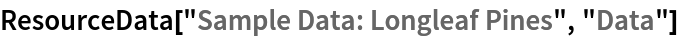Out=Summary of the spatial point data:

 In:=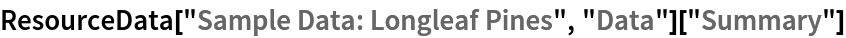Out=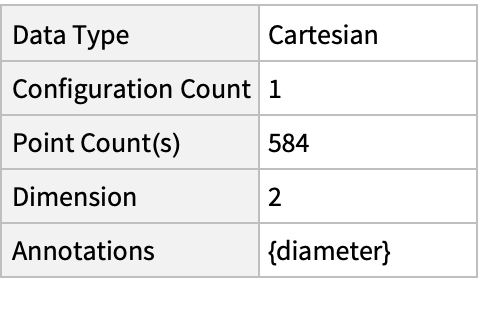### Visualizations (3)

Plot the points:

 In:=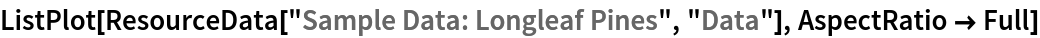Out=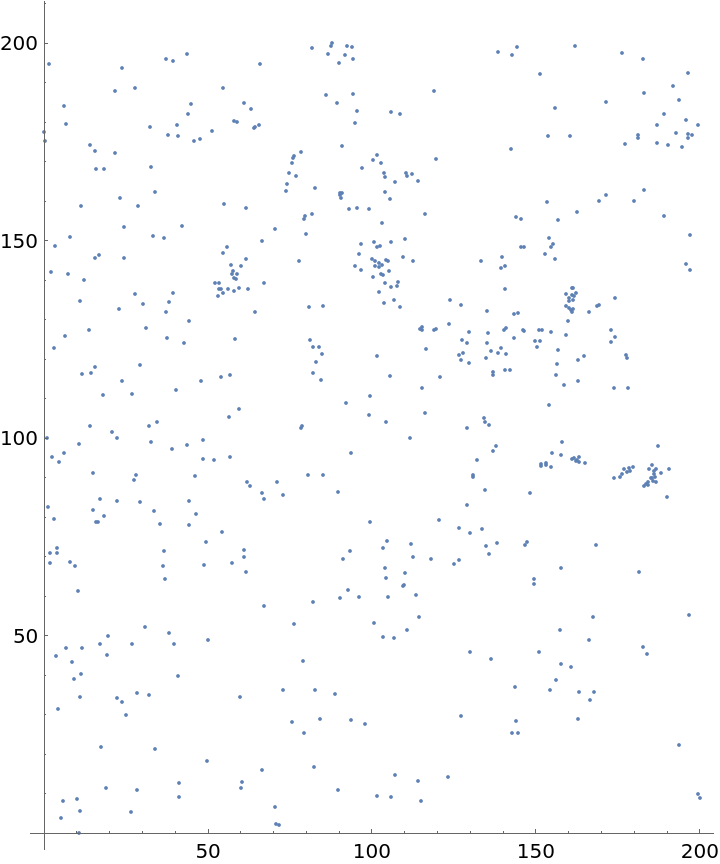Plot the spatial point data with diameter marks:

 In:=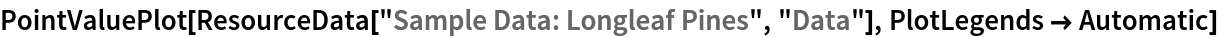Out=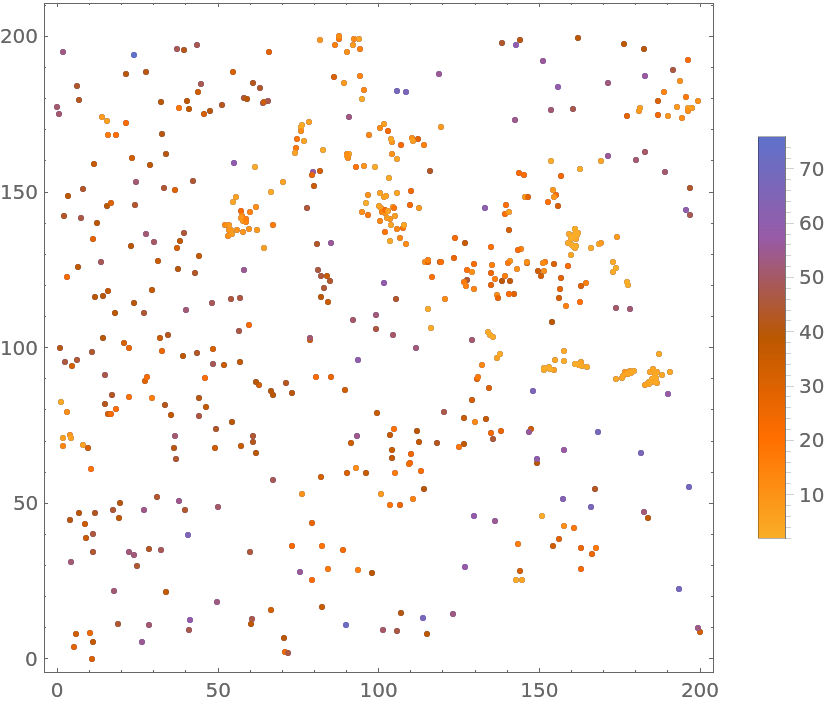Visualize the smooth point density:

 In:=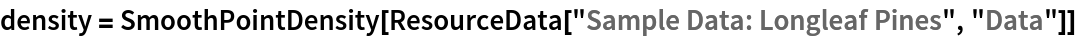Out=In:=Out=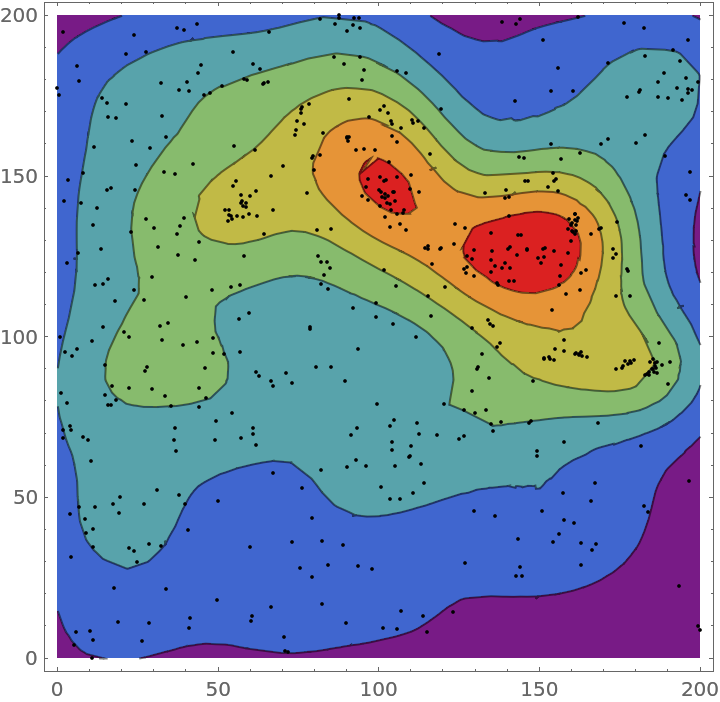### Analysis (3)

Compute probability of finding a point within given radius of an existing point - NearestNeighborG is the CDF of the nearest neighbor distribution:

 In:=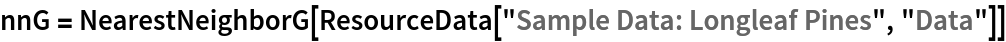Out=In:=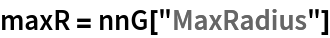Out=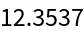In:=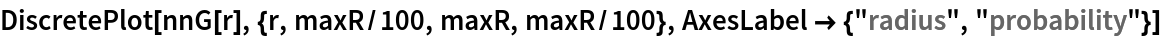Out=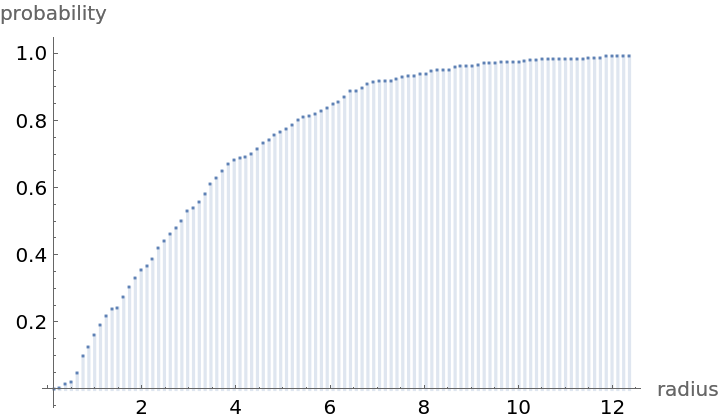Mean distance between a typical point and its nearest neighbor (for positive support distribution can be approximated via a Riemann sum of 1-CDF):

 In:=In:=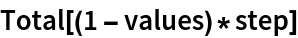Out=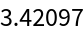Test for complete spacial randomness:

 In:=Out=Gosia Konwerska, "Sample Data: Longleaf Pines" from the Wolfram Data Repository (2021)